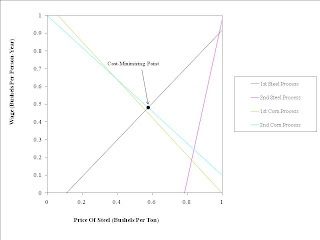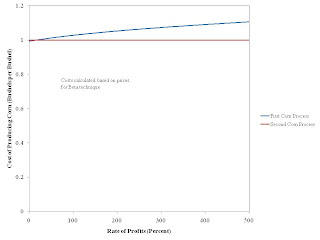## Friday, March 11, 2011

### Three Routes To The Choice Of Technique

1.0 Introduction
I think the analysis of the choice of technique in a steady state is a settled question. (The meaning of Sraffa's equations in wider contexts can be debated.) One strength of the analysis of the choice of technique is the existence of several methods of analysis, all reaching the same conclusion. If one wanted to overthrow this analysis, one would need to show that one is not attacking just one such method, but all of them - or at least as many as possible. This post illustrates this strength of the analysis by presenting three such methods.

2.0 Example Technology
I need an example technology (Table 1) to use in stepping through different methods for analyzing the choice of technique. Each process requires the inputs shown to be purchased at the start of the production period (a year) for each unit of output produced and available at the end of the year. Two processes are known for producing steel, and two other processes are likewise known for producing corn. The coefficients are fairly arbitrary. In this example, to produce any net output in a steady state, all commodities - that is, both steel and corn - must be produced.
 Inputs Industry Sector Steel Industry Corn Industry FirstSteel-ProducingProcess SecondSteel-ProducingProcess FirstCorn-ProducingProcess SecondCorn-ProducingProcess Labor (Person-Yrs) 3220/3321 13930/63099 3115/3321 1 Steel (Tons) 0 0 1/2 9/20 Corn (Bushels) 1/18 2752/7011 0 0 Output 1 Ton 1 Ton 1 Bushel 1 Bushel
The analysis of the choice of technique calculates which production process would be adopted for each combination of prices and interest rates. For this technology to be compatible with a steady state, at least one process for producing steel and one process for producing corn must be adopted. A "technique" consists of one process from each of the industries in this example. Table 2 defines the four techniques, each named with a greek letter. (I think this convention of using greek letters in this context may have been introduced by Joan Robinson.)
Table 2: Techniques and Production Processes
TechniqueSteel-Producing
Process
Corn-Producing
Process
AlphaFirstFirst
BetaFirstSecond
GammaSecondFirst
DeltaSecondSecond
A technique, in this case, is expressed by a 2-element row vector of direct labor coefficients and a square Leontief Input-Output matrix. For example, the labor coefficients, a0α, for the first technique are:
a0α = [(3220/3321) (3115/3321)]
The Leontief Input-Output matrix, Aα, for the first technique can be expressed as two columns a.1α and a.2α:
Aα = [ a.1α a.2α]
The first labor coefficient and the first column in the Leontief Input-Output matrix come from the specified production process from the steel industry for that technique:
a.1α = a.1β = [0, (1/18)]T
The second labor coefficient and the second column in the Leontief Input-Output matrix come from the specified production process from the corn industry for that technique:
a.2α = a.2γ = [(1/2), 0]T
I leave to the reader how to completely specify a0β, Aβ, a0γ, Aγ, a0δ, and Aδ.

3.0 Direct Method
Heinz D. Kurz and Neri Salvadori refer to this method for analyzing the choice of technique I describe here as the "Direct Method". Before proceeding, I need to introduce some notation. Let p be a two-element row vector of prices:
p = [p1, p2]
where p1 is the price of a ton steel and p2 is price of a bushel corn. Let w be the wage, assumed to be paid at the end of the year for each person-year of labor expended during the year. Let r be the rate of interest, also called the rate of profits.

I need to introduce a column vector to represent the numeraire. Let e2 be the second column of the 2x2 identity matrix:
e2 = [0, 1]T
The assumption that e2 is the numeraire implies the following equation:
p e2 = 1
This specification of the numeraite implies that, p2, the price of a bushel corn is unity.

The problem is to find a pair (p, w), given the interest rate r, such that
• No process can be operated with costs less than revenues.
• For any process that is operated, the costs do not exceed the revenues.
The first condition implies the following four inequalities must hold:
p a.1α(1 + r) + a01α wp1
p a.1γ(1 + r) + a01γ wp1
p a.2α(1 + r) + a02α wp2
p a.2β(1 + r) + a02β wp2
The conjunction of the requirement that steel be produced with the second condition implies that one of the first two inequalities must be met with a strict equality. The analogous requirement for corn production implies that at least one of the last two inequalities must be met with equality.

These specifications are easily graphed (Figure 1). Given the interest rate, the first two inequalities yield upward-sloping lines in the the figure. The last two inequalities yield the downward-sloping lines. The first condition implies the solution must lie on or above all of the lines in the figure. The second condition implies that the solution must lie on
• At least one of the upward-sloping lines
• At least one of the downward-sloping lines.
The only point that satisfies these conditions is graphed. It lies on the upward-sloping line corresponding to the first steel-producing process and the downward-sloping line corresponding to the second corn-producing process. Thus, this analysis shows that the beta technique is cost-minimizing at an interest rate of 100%. The solution wage and price of steel can be read off the figure.Figure 1: Direct Method Illustrated At r = 100%
The direct method is easily generalized to any finite number of techniques. Each additional production process results in an additional line in the figure, upward-sloping for the steel industry and downward-sloping for the corn industry. The method also generalizes for any finite number of commodities. Each additional commodity results in the introduction of another dimension to the figure. Although the figure quickly becomes unvisualizable, the mathematics generalizes.

4.0 Indirect Method
The indirect method generalizes to cases in which an uncountably infinite number of techniques are available. It is based on constructing the wage-rate of profits frontier as the outer envelope of the wage-rate of profits curve for each technique (Figure 2). I illustrate how to construct the wage-rate of profits curve for the Alpha technique.Figure 2: Indirect Method Illustrated

The condition that the same rate of profits be earned for each process comprising a technique yields a system of two equations:
p Aα(1 + r) + a0α w = p
One also has the equation setting the price of the numeraire to unity:
p e2 = 1
For a given interest rate r, the above is a linear system of three equations for three variables (p1, p2, and w). One can solve the system to express each of these three variables as a function of the interest rate. The wage, for example, can be found as:
w = 1/(a0α [ I - (1 + r)Aα]-1e2)
One knows, from a theorem due to Perron and Frobenius, that the inverse exists between an interest rate of zero and some maximum interest rate.

Figure 2 shows the wage-rate of profits curves for each of the four techniques. The cost-minimizing technique at each rate of interest is the one with the highest wage. Points at which the rate-rate of profits curves for two or more techniques interesect on the outer frontier are known as switch points. The two switch points are shown in the example. The Gamma technique is cost-minimizing for a very low interest rate. For a somewhat larger interest rate, the Delta technique is cost-minimizing. Finally, the Beta technique is cost minimizing for larger interest rates. My exposition illustrates that the direct and indirect methods give the same conclusion. For example, the wage-rate of profits frontier shows that the Beta technique is cost-minimizing for an interest rate of 100%.

5.0 Cost Minimization Algorithm
This method I take from J. E. Woods. He provides an algorithm for finding the cost-minimizing technique(s), given the interest rate.
1. Pick an initial technique. (For illustration, I start with the Beta technique in the example.)
2. Solve the equations specifying the wage-rate of profits curve for the selected technique. So you now have a price vector p and the wage w.
3. Using p and w, calculate the cost of producing a ton steel with each of the known production processes (Figure 3).
4. If the steel-producing process in the selected technique is cheapest, go to Step 6. Otherwise go to Step 5. (In the example, one would go to Step 5 for low interest rates and to Step 5 for higher interest rates.
5. Replace the steel-producing process in the technique analysed in Step 2 with the cheapest steel-producing process identified in Step 4. Solve the equations specifying the wage-rate of profits curve for the newly selected technique. Use the resulting p and w in Step 6. (In the example, one would calculate the wage-rate of profits curve for the Delta technique for a sufficiently low interest rate.)
6. Using the specified p and w, calculate the cost of producing a bushel corn with each of the known production processes (Figure 4).
7. If cost of producing corn could be found in Step 6 for the technique selected in Step 2 and the corn-producing process in that technique is cheapest, then stop. You have identified the cost-minimizing technique. Otherwise, replace the corn-producing process in the technique analyzed in Step 6 with the cheapest corn-producing process identified in Step 6. (In the example, the algorithm would terminate in one-pass for a sufficiently high interest rate, with the Beta technique identified as the cost-minimizing technique.)
8. Go to Step 2.Figure 3: Costs of Producing Steel with Prices for the Beta TechniqueFigure 4: Costs of Producing Corn with Prices for the Beta Technique
Figures 3 and 4 suggest that for a sufficiently low interest rate, the technique consisting of the second steel-producing process and the first corn-producing process, that is, the Gamma technique, is cost-minimizing. For a somewhat higher interest rate, the technique consisting of the second steel-producing process and the second corn-producing process, that is, the Delta technique, seems to be cost-minimizing. And, as noted above, the algorithm terminates with the Beta technique identified as the cost-minimizing technique at an even higher interest rate. In other words, the graphs suggest that the above algorithm converges to the same solution as the indirect method.

6.0 Conclusions
I have not exhausted the methods available for analyzing the choice of technique. For example, I have not formulated any Linear Programs above. Nor have I presented the diagram in my 2005 Manchester School paper. Furthermore, I have glossed over many interesting mathematical questions, such as proving the existence of solutions and proving that all methods give the same result. But this post is already too long.

References
• Heinz D. Kurz and Neri Salvadori (1995) Theory of Production: A Long-Period Analysis. Cambridge University Press.
• J. E. Woods (1990) The Production of Commodities: An Introduction to Sraffa, Humanities Press.